# CBSEtips.in

## Wednesday 24 February 2021

### CBSE Class 8 Maths - MCQ and Online Tests - Unit 5 - Data Handling

#### CBSE Class 8 Maths – MCQ and Online Tests –  Unit 5 – Data Handling

Every year CBSE students attend Annual Assessment exams for 6,7,8,9,11th standards. These exams are very competitive to all the students. So our website provides online tests for all the 6,7,8,9,11th standards’ subjects. These tests are also very effective and useful for those who preparing for any competitive exams like Olympiad etc. It can boost their preparation level and confidence level by attempting these chapter wise online tests.

These online tests are based on latest CBSE syllabus. While attempting these, our students can identify their weak lessons and continuously practice those lessons for attaining high marks. It also helps to revise the NCERT textbooks thoroughly

#### CBSE Class 8 Maths – MCQ and Online Tests – Unit 5 – Data Handling

I. Observe the following bar graph carefully and answer the following questions :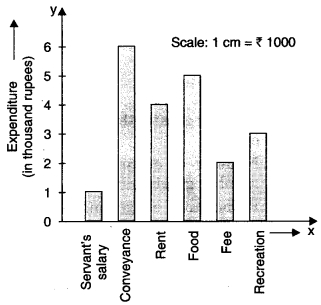Question 1.
Rs 5000 is the expenditure done on
(a) rent
(b) food
(c) fee
(d) recreation.

Hint:
$$\frac{5000}{1000}$$ = 5 cm is the height of the bar corresponding to food.

Question 2.
On which item has the minimum expenditure been done ?
(a) Servant’s salary
(b) Food
(c) Rent
(d) Conveyance.

Hint:
The height of the bar corresponding to servant’s salary is minimum.

Question 3.
What is the expenditure done on food ?
(a) Rs 1000
(b) Rs 2000
(c) Rs 3000
(d) Rs 5000.

Hint:
Expenditure done on food = 5 × 1000
= Rs 5000.

Question 4.
On which item has the maximum expenditure been done ?
(a) Conveyance
(b) Rent
(c) Fee
(d) Servant’s salary.

Hint:
The height of the bar corresponding to conveyance is the maximum.

Question 5.
What is the difference of expenditures done on conveyance and rent ?
(a) Rs 1000
(b) Rs 2000
(c) Rs 3000
(d) Rs 4000.

Hint:
Expenditure done on conveyance
= 6 × 1000 = Rs 6000
Expenditure done on rent = 4 × 1000
= Rs 4000
? Difference = Rs 6000 – Rs 4000
= Rs 2000.

Question 6.
Rs 6000 is the expenditure done on
(a) fee
(b) rent
(c) conveyance
(d) food.

Hint:
$$\frac{6000}{1000}$$ = 6 cm is the height of the bar corresponding to conveyance.

Question 7.
How much expenditure has been done in all ?
(a) Rs 21000
(b) Rs 18000
(c) Rs 15000
(d) Rs 20000.

Hint:
Total expenditure
= (1 + 6 + 4 + 5 + 2 + 3) × 1000 = Rs 21000

II. Observe the following bar graph carefully and answer the following questions :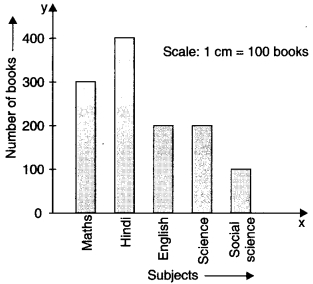Question 1.
Of which subject are there the maximum books ?
(a) Hindi
(b) English
(c) Maths
(d) Science.

Hint:
The height of the bar corresponding to Hindi is maximum.

Question 2.
Of which subject are there the minimum books ?
(a) Social Science
(b) Hindi
(c) English
(d) Science.

Hint:
The height of the bar corresponding to Social Science is minimum.

Question 3.
How many books are there of the subject whose books are maximum ?
(a) 100
(b) 200
(c) 300
(d) 400.

Hint:
Hindi ? 400.

Question 4.
How many books are there of the subject whose books are minimum ?
(a) 100
(b) 200
(c) 300
(d) 400.

Hint:
Social Science ? 100.

Question 5.
300 books are of the subject
(a) Maths
(b) English
(c) Hindi
(d) Science.

Hint:
300 ? Maths.

Question 6.
Which two subjects have the same number of books ?
(a) Maths and Hindi
(b) Hindi and English
(c) English and Science
(d) Science and Social Science.

Hint:
English ? 200
Science ? 200

Question 7.
The difference of the number of books of English and Science is
(a) 200
(b) 100
(c) 400
(d) 0.

Hint:
200 – 200 = 0.

Question 8.
The difference of the number of books of Hindi and Social Science is
(a) 200
(b) 300
(c) 400
(d) 100.

Hint:
400 – 100 = 300.

Question 9.
The total number of books is
(a) 1200
(b) 1400
(c) 1600
(d) 1800.

Hint:
300 + 400 + 200 + 200 + 100 = 1200.

Question 10.
The total of the number of books of English and Science is
(a) 200
(b) 100
(c) 400
(d) 0.

Hint:
200 + 200 = 400.

III. Study the following frequency distribution table and answer the questions given below :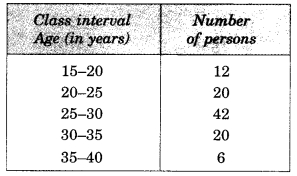Question 1.
Which two classes have the same frequency ?
(?) 15-20 and 35-40
(b) 20-25 and 30-35
(c) 15-20 and 30-35
(d) 20-25 and 25-30.

Hint:
Frequency of class 20-25 = Frequency of class 30-35 = 20.

Question 2.
Which class has the highest frequency ?
(a) 15-20
(b)20-25
(c) 25-30
(d) 35-40.

Hint:
Highest frequency = 42 ? 25 – 30.

Question 3.
The difference between the frequencies of the class intervals 20-25 and 30-35 is
(a) 0
(b) 10
(c) 20
(d) 5.

Hint:
Frequency of class 20-25 = 20.
Frequency of class 30-35 = 20
? Difference = 20-20 = 0.

Question 4.
Which class has the lowest frequency ?
(a) 35-40
(b)30-35
(c) 15-20
(d) 25-30.

Hint:
Lowest frequency = 6 ? 35 – 40.

Question 5.
What is the size of the class intervals ?
(a) 5
(b) 10
(c) 15
(d) 20.

Hint:
Size = 20 – 15 = 5.

Question 6.
What is the upper limit of the class interval 25-30 ?
(a) 20
(b)25
(c) 30
(d) 35.

Question 7.
What is the lower limit of the class interval 35-40 ?
(a) 20
(b) 25
(c) 30
(d) 35.

IV. Study the following frequency distribution table and answer the questions given below :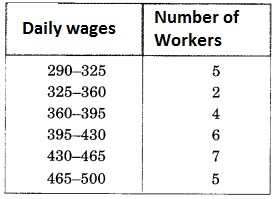Question 1.
The frequency of the third class is
(a) 5
(b) 4
(c) 2
(d) 7.

Hint:
Third class is 360 – 395.

Question 2.
The lower limit of the sixth class is
(a) 395
(b) 430
(c) 465
(d) 360.

Hint:
Sixth class is 465 – 500.

Question 3.
The number of workers getting daily wages 395-430 (in ?) is
(a) 4
(b) 5
(c) 6
(d) 7.

Hint:
Frequency of class 395 – 430 is 6.

Question 4.
The size of the class intervals is
(a) 25
(b) 30
(c) 40
(d) 35.

Hint:
Fourth class is 395-430.

Question 5.
The upper limit of the fourth class is
(a) 430
(b) 395
(c) 465
(d) 500.

Hint:
Size = 325 – 290 = 35.

Question 6.
Which two classes have the same frequency ?
(a) 290-325 and 465-500
(b) 290-325 and 325-360
(c) 430-465 and 465-500
(d) 325-360 and 360-395.

Hint:
Frequency of class 290-325
= Frequency of class 465-500 = 5.

Question 7.
The class with highest frequency is
(a) 430-465
(b) 465-500
(c) 395-430
(d) 290-325.

Hint:
Highest frequency = 7 ? 430-465.

Question 8.
The class with lowest frequency is
(a) 325-360
(b) 360-395
(c) 465-500
(d) 395-430.

Hint:
Lowest frequency = 2 ? 325-360.

Question 9.
The number of workers getting wages ? 395 and above is
(a) 18
(b) 24
(c) 12
(d) 28.

Hint:
Required number = 6 + 7 + 5 = 18.

Question 10.
The number of workers getting wages below ? 360 is
(a) 7
(b) 6
(c) 5
(d) 4.

Hint:
Required number = 5 + 2 = 7.

Question 11.
The total number of workers is
(a) 29
(b) 22
(c) 28
(d) 21.

Hint:
Required number = 5 + 2 + 4 + 6 + 7 + 5
= 29.

V. Observe the histogram and answer the questions given below :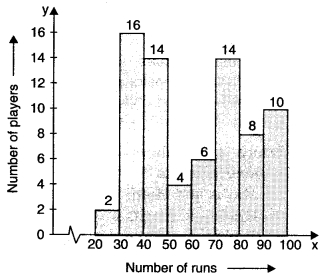Question 1.
Which group contains maximum players ?
(a) 20-30
(b) 30-40
(c) 40-50
(d) 70-80.

Hint:
Maximum frequency = 16 ? 30-40.

Question 2.
Which group has minimum players ?
(a) 20-30
(b) 50-60
(c) 60-70
(d) 30-40.

Hint:
Minimum frequency = 2 ? 20-30.

Question 3.
Which two groups have the same number of players ?
(a) 30-40 and 40-50
(b) 20-30 and 30-40
(c) 40-50 and 70-80
(d) 80-90 and 90-100.

Hint:
Frequency of group 40-50 = 14
= Frequency of group 70-80.

Question 4.
How many players make runs 80 and above ?
(a) 2
(b) 8
(c) 10
(d) 18.

Hint:
Required number = 8 + 10 = 18.

Question 5.
How many players make runs less than 40?
(a) 8
(b) 2
(c) 18
(d) 10.

Hint:
Required number = 2 + 16 = 18.

Question 6.
How many players make runs 50 to less than 60 ?
(a) 1
(b) 2
(c) 3
(d) 4

Hint:
Required number
= Frequency of group 50-60 = 4.

Question 7.
The total number of players making runs 20 and more is
(a) 74
(b) 64
(c) 84
(d) 54.

Hint:
Required number
= 2 + 16 + 14 + 4 + 6 + 14 + 8 + 10 = 74.

VI. Observe the histogram and answer the questions given below :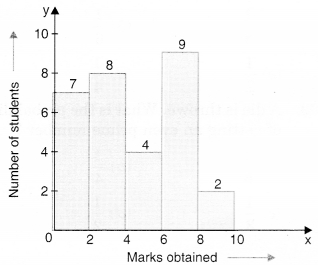Question 1.
The number of students getting marks 4 to less than 6 is
(a) 2
(b) 4
(c) 6
(d) 8.

Hint:
Required number
= Frequency of class (4 – 6) = 4.

Question 2.
Which groups contain the maximum number of students ?
(a) 2-4
(b) 4-6
(c) 6-8
(d) 8-10.

Hint:
Maximum number of students
= 9 ? 6 – 8.

Question 3.
The number of students getting marks in the groups 4-6 or 8-10 is
(a) 2
(b) 4
(c) 6
(d) 8.

Hint:
Required number = 4 + 2 = 6.

Question 4.
Which group contains the minimum number of students ?
(a) 0-2
(b) 2-4
(c) 6-8
(d) 8-10.

Hint:
Minimum number of students
= 2 ? 8 -10.

Question 5.
The total number of students is
(a) 10
(b) 20
(c) 25
(d) 30.

Hint:
Total number of students
= 7 + 8 + 4 + 9 + 2 = 30.

Question 6.
The number of students getting marks 6 and above is
(a) 11
(b) 10
(c) 12
(d) 9.

Hint:
Required number = 9 + 2 = 11.

Question 7.
The number of students getting marks less than 4 is
(a) 10
(b) 15
(c) 7
(d) 8

Hint:
Required number = 7 + 8 = 15.

Question 8.
The number of students getting marks 6-8 is greater than the number of students getting marks 2-4 by
(a) 1
(b) 2
(c) 3
(d) 4.

Hint:
Required difference = 9 – 8 = 1.

VII. Observe the pie chart given below and answer the following questions: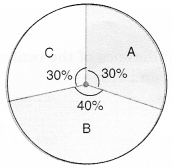Question 1.
What is the difference between the central angles for sector B and sector C ?
(a) 36°
(b) 72°
(c) 9°
(d) 81°.

Hint:
144° – 108° = 36°.

Question 2.
The central angle for sector A is
(a) 108°
(b) 144°
(c) 72°
(d) 150°.

Hint:
Central angle for sector A
= $$\frac{30}{100}$$ × 360° = 108°.

Question 3.
The central angle for sector B is
(a) 108°
(b) 144°
(c) 72°
(d) 120°.

Hint:
Central angle for sector B
= $$\frac{40}{100}$$ × 360° = 144°.

Question 4.
Which sector has the greatest angle ?
(a) A
(b) B
(c) C
(d) None of these.

Hint:
Greatest percentage = 40% ? B.

VII. Observe the pie chart and answer the following questions :Question 1.
The difference of the central angles for green and blue is
(a) 45°
(b) 90°
(c) 180°
(d) 22$$\frac{1°}{2}$$

Hint:
Central angle for blue = 180°
Central angle for green = 90°
? Difference = 180° – 90° = 90°.

Question 2.
Which colour has the greatest central angle ?
(a) Red
(b) Yellow
(c) Green
(d)Blue.

Hint:
Blue ? 180°.

Question 3.
Which two colours have the same central angles ?
(a) Red, yellow
(b) Red, green
(c) Yellow, green
(d) Blue, red.

Hint:
Red ? 45%
Yellow ? 45%.

Question 4.
A child has a block in the shape of a cube with one letter written on each face as shown below:The cube is thrown once. What is the probability of getting A ?
(a) $$\frac{1}{3}$$
(b) $$\frac{1}{6}$$
(c) $$\frac{1}{2}$$
(d) $$\frac{1}{4}$$

Hint:
Probability = $$\frac{2}{6}$$ = $$\frac{1}{3}$$

Question 5.
A die is thrown. What is the probability of getting an even prime number ?
(a) $$\frac{1}{6}$$
(b) $$\frac{1}{4}$$
(c) $$\frac{1}{3}$$
(d) $$\frac{1}{2}$$

Hint:
Even prime number = 2
? Probability = $$\frac{1}{6}$$

Question 6.
The proportion of sector for red is
(a) $$\frac{1}{2}$$
(b) $$\frac{1}{4}$$
(c) $$\frac{1}{8}$$
(d) $$\frac{1}{3}$$

Hint:
$$\frac{45°}{360°}$$ = $$\frac{1}{8}$$

Share: